CBSE Class 10 Sample Paper for 2019 Boards

Class 10
Solutions of Sample Papers for Class 10 Boards

Question 21 (OR 2 nd   question)

A solid sphere of radius 3 cm is melted and then recast into small spherical balls each of diameter 0.6 cm. Find the number of balls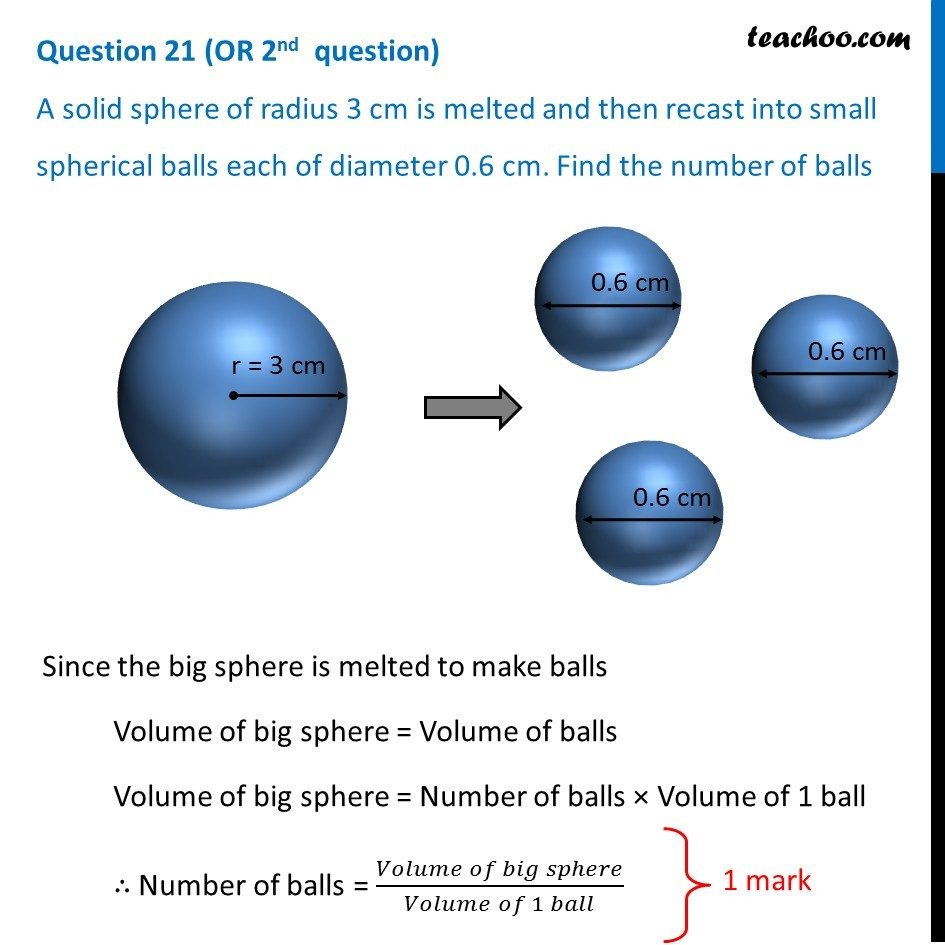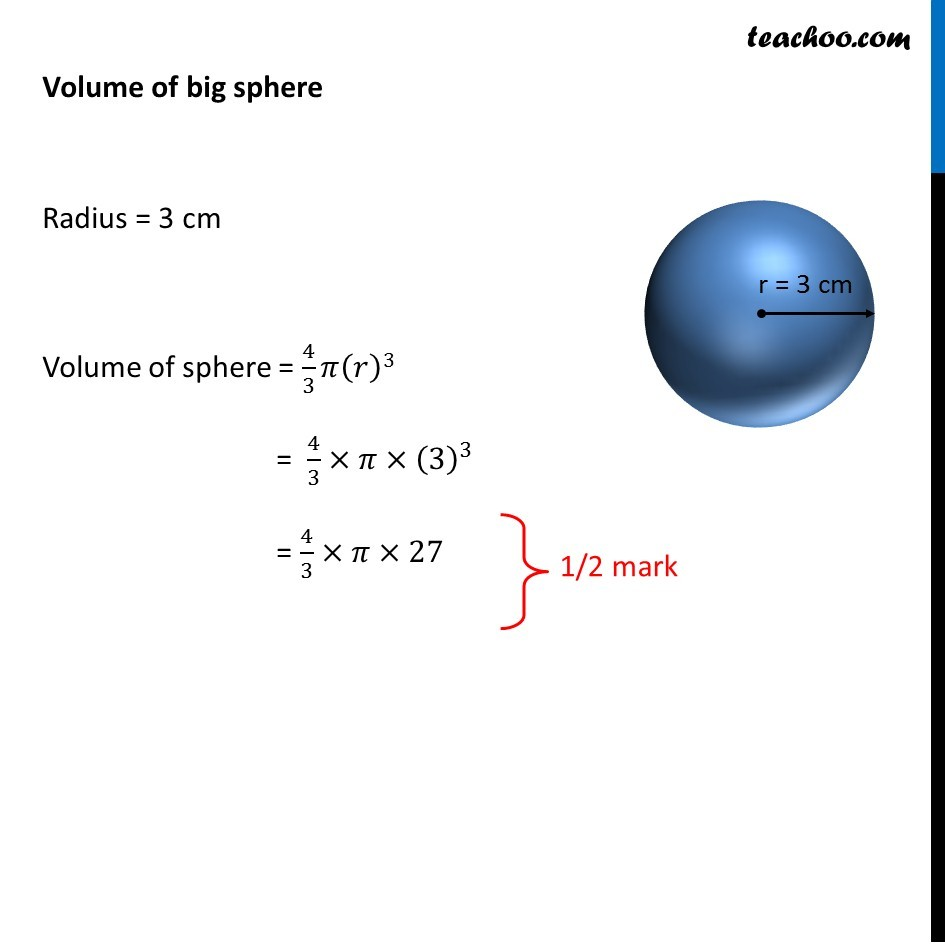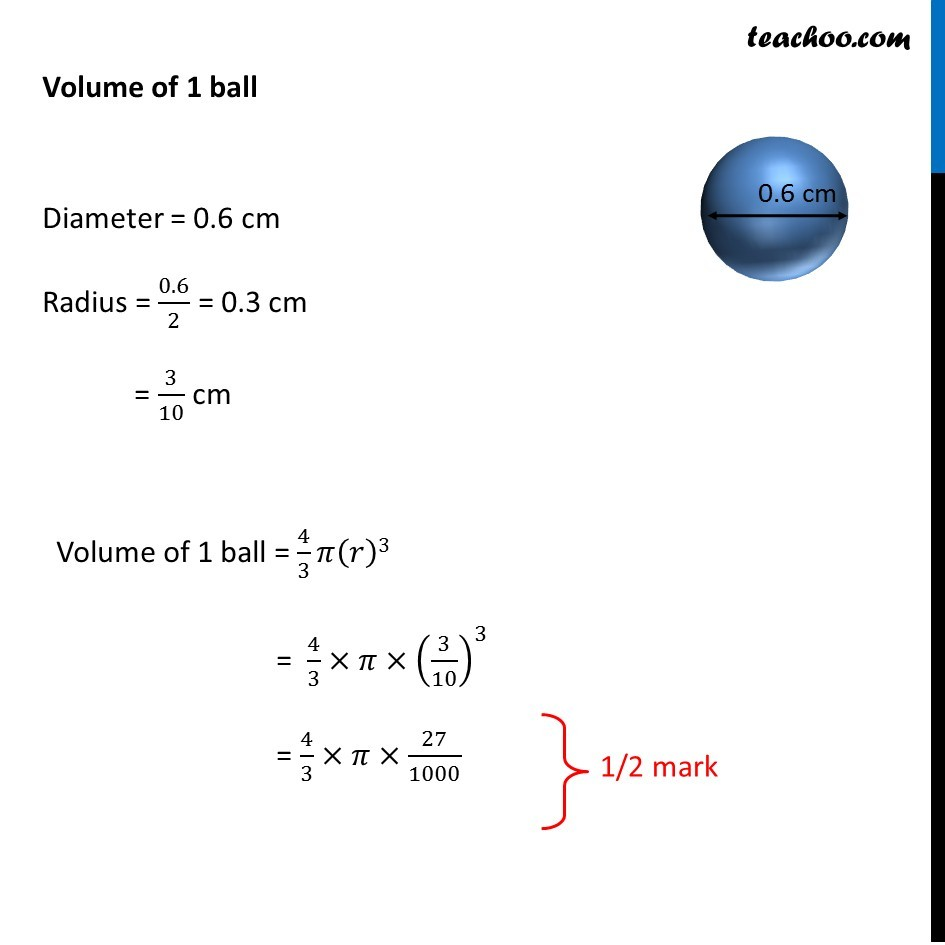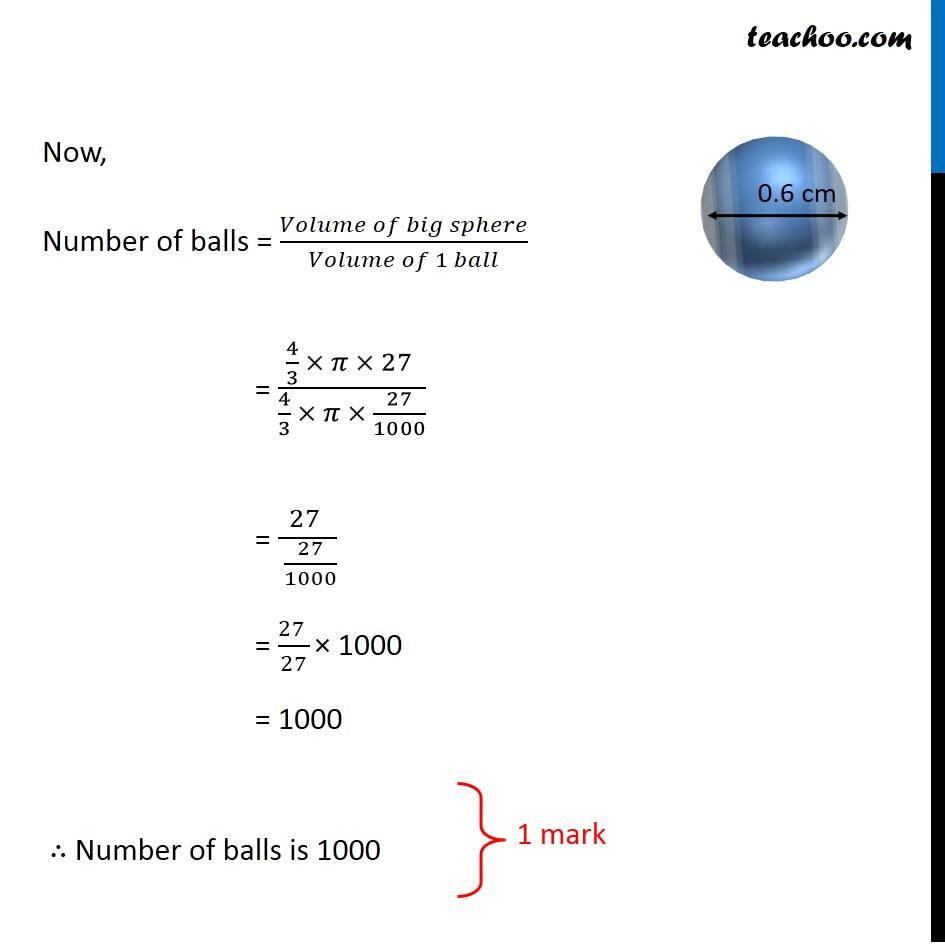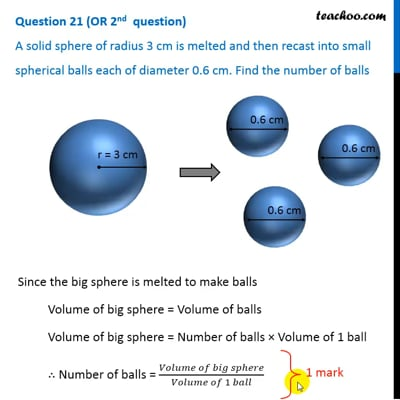This video is only available for Teachoo black users

### Transcript

Question 21 (OR 2nd question) A solid sphere of radius 3 cm is melted and then recast into small spherical balls each of diameter 0.6 cm. Find the number of balls Since the big sphere is melted to make balls Volume of big sphere = Volume of balls Volume of big sphere = Number of balls × Volume of 1 ball ∴ Number of balls = (𝑉𝑜𝑙𝑢𝑚𝑒 𝑜𝑓 𝑏𝑖𝑔 𝑠𝑝ℎ𝑒𝑟𝑒)/(𝑉𝑜𝑙𝑢𝑚𝑒 𝑜𝑓 1 𝑏𝑎𝑙𝑙) Volume of big sphere Radius = 3 cm Volume of sphere = 4/3 𝜋(𝑟)3 = 4/3×𝜋×(3)^3 = 4/3×𝜋×27 Volume of 1 ball Diameter = 0.6 cm Radius = 0.6/2 = 0.3 cm = 3/10 cm Volume of 1 ball = 4/3 𝜋(𝑟)3 = 4/3×𝜋×(3/10)^3 = 4/3×𝜋×27/1000 Now, Number of balls = (𝑉𝑜𝑙𝑢𝑚𝑒 𝑜𝑓 𝑏𝑖𝑔 𝑠𝑝ℎ𝑒𝑟𝑒)/(𝑉𝑜𝑙𝑢𝑚𝑒 𝑜𝑓 1 𝑏𝑎𝑙𝑙) = (4/3 × 𝜋 × 27" " )/(4/3 × 𝜋 × 27/1000) = 27" " /( 27/1000) = 27" " /27 × 1000 = 1000 ∴ Number of balls is 1000